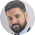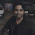# Unitary method - Concept and Problems

Today I am going to discuss Unitary Method under this section. Unitary method is part of Ratio and Proportion chapter. This topic has various applications and can be used in many other topics like Time and Work. It involves basic calculations and generally includes two types of proportions: Direct proportion and Indirect proportion. In unitary method question we just need to identify whether it is a direct proportionality or indirect proportionality. Rest is very simple task.

Unitary Method: Finding value of one unit first. Then finding value of required number of units. Therefore, Unitary method comprises two following steps:

Step 1 = Find the value of one unit.

Step 2 = Then find the value of required number of units.
For example: If 1 mango = Rs 5, then 5 mangoes = 5*5 = Rs.25

## Problems for practice

Problem1: If 6 note books costs Rs.45, then how much 8 note books costs?

Solution: 6 notebooks = Rs.45
1 notebook = Rs.45/ 6 ( unit price)
8 notebooks = Rs. (45/ 6)*8 = Rs.60

Problem 2: 85 students consume a particular stock in 60 days. How many days 51 students will take to consume that stock?

Solution: This is a case of inverse proportion.

More students, less days ; Less students, more days.

85 students consume in 60 days. Lets write it as:
85 students = 60 days

Converting it into a unit
1 student = 85 * 60 days ( less students, more days)

Therefore, 51 students = (85 * 60)/ 51 days
⇒ 51 students = 5100/ 51 = 100 days
⇒ 51 students consume that stock in 100 days.

Problem 3: 5 workers can complete work in 20 days. In how many days, 10 workers can complete the work?

Solution: This is a case of inverse proportion.

More workers, less days; less workers, more days

5 workers complete work in 20 days
i.e. 5 workers = 20 days

1 worker = 20 * 5 days (less workers, more days)

Therefore,10 workers = 110/ 10 = 10 days
⇒ 10 workers take 10 days to complete work.

## Problems related to three variables

Problem1: 40 persons consume 200kg rice in 30 days. In how many days will 3 persons consume 500kg rice?

Solution: In problems related to three variables, we will firstly deal with two variables then with the third.

According to question, 40 persons consume 200 kg rice in 30days

Firstly, I'm going to deal with quantity of rice and number of days

40 PERSONS:
200 kg = 30 days
1 kg = 30/ 200 days (Direct proportion)
500 kg = (30/ 200) * 500 = 75 days

Now, i will deal with third variable

1 PERSON:
40 persons = 75 days
1 person = 75* 40 days ( Inverse proportion)

30 PERSONS:
30 PERSONS = (75* 40)/ 30
⇒ 3000/30 = 100 days

Therefore, 30 persons consume 500kg in 100 days.

Problem2: 500 persons consume 100 kg of wheat in 20 days. How many days will 20 persons take to consume 1000 kg rice?

Solution: Do it yourself#### What's trending in BankExamsToday

Smart Prep Kit for Banking Exams by Ramandeep Singh - Download here

##### Join 40,000+ readers and get free notes in your email1.thank u very much for the tricks..can u send short tricks of LCM and hcf on my id

1.2.thank u so much sir. it is very helpful for me

3.sir, ans of last problem question is ?

1.I request you to use your name. I don't know which problem you are talking about !

2.THE ANSWER IS 125000 DAYS, I DONOT KNOW WETHER IT IS CORRECT OR NOT.

4.Dear i have only one month to prepare ibps clerk can i make it.............im not so good in maths and reasoning......kindly guide me.......

1.Go through previous articles, wrote lots of tips and tricks

5.Please post some more shortcuts!!!!

6.Problem1.Question is 3 but U got it for 30persons but thanks for the solving strategy

7.sir please guide me for di because it is the toughest section as per all sessions of sbi po ... can you please give me tricks for di with more complexity.

8.sir i am referring your posts for my sbi po preparation .. thank you so much for your kind effort.. my exam will be held on 29th june .. i am worried about di tricky questions with 3 or more varaibles in tables and line graphs .. please provide some tricks and advice me for last week preparation.. i will be highly thankful to u ..

1.I have shared many questions in my recent posts, please check

9.Hi,
Kindly explain this question-
1200 soldiers in a fort had enough food for 28 days. After 4 days, some soldiers were sent to another fort and thus, the food lasted for 32 more days. How many soldiers left the fort?

Thanks.

Thanks for commenting. Follow us on Telegram. Search BankExamsToday